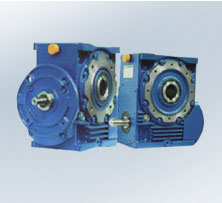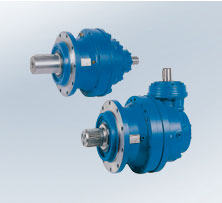# Rossi Gearmotors

Gear Reducers products list Parallel and right angle shaft gear reducers (MN2 = 7 100 daN m)

• Parallel and right angle shaft gear reducers (MN2 90 ... 400 kN m)
• Worm gear reducers
• Planetary gear reducers
• Coaxial gear reducers
• Shaft mounted gear reducers
• Right angle shaft gear reducers

Parallel and right angle shaft gear reducers
(MN2 = 7 100 daN m)
17 Sizes (50 ... 360)
Nominal power PN2 0,212 ÷ 2 700 kW
Nominal torque MN2 = 7 100 daN m
Transmission ratio iN 2,5 ... 12 500

Parallel and right angle shaft gear reducers
(MN2 90 ... 400 kN m)
10 Sizes (400 ... 631)
Nominal power PN2 16 ÷ 3 650 kW
Nominal torque MN2 90 ÷ 400 kN m
Transmission ratio iN 8 ... 315

Worm gear reducers
14 Sizes (32 ... 250)
Nominal power PN2 0,01 ÷ 67 kW
Nominal torque MN2 = 1 900 daN m
Transmission ratio iN 10 ... 16 000

Planetary gear reducers
16 Sizes (200 ... 696)
Nominal power PN2 0,046 ÷ 560 kW
Nominal torque MN2 = 20 000 daN m
Transmission ratio iN 12,5 ... 3 000

Coaxial gear reducers 16 Sizes (32 ... 180)
Nominal power PN2 0,082 ÷ 117 kW
Nominal torque MN2 = 1 000 daN m
Transmission ratio iN 6,3 ... 6 300

Shaft mounted gear reducers
7 Sizes
Nominal power PN2 0,6 ÷ 85 kW
Nominal torque MN2 = 1 180 daN m
Transmission ratio iN 10 ... 25

Right angle shaft gear reducers
7 Sizes (80 ... 320)
Nominal power PN2 0,16 ÷ 500 kW
Nominal torque MN2 = 600 daN m
Transmission ratio i 1 ... 6,25

Gearmotors products list Worm gearmotors

• STANDARDFIT Worm gearmotors NEW
• Coaxial gearmotors
• STANDARDFIT Coaxial gearmotors NEW
• Parallel and right angle shaft gearmotors
• Planetary gearmotors
• Synchronous and asynchronous servogearmotors
• Integrated low backlash planetary servogearmotors
• Gearmotors for roller ways
• D.C. gearmotors

Worm gearmotors
14 Sizes (32 ... 250)
Power P1 0,09 ... 55 kW
Nominal torque MN2 = 1 900 daN m
Transmission ratio iN 7,1 ... 16 000

STANDARDFIT Worm gearmotors
6 Sizes (118 ... 742)
Power P1 0,09 ... 5,5 kW
Nominal torque MN2 = 670 daN m
Transmission ratio iN 6 ... 75

Coaxial gearmotors
16 Sizes (32 ... 180)
Power P1 0,09 ... 75 kW
Nominal torque MN2 = 1 000 daN m
Transmission ratio iN 4 ... 6 300

STANDARDFIT Coaxial gearmotors
8 Sizes (0 ... 7)
Power P1 0,09 ... 11 kW
Nominal torque MN2 = 900 daN m
Transmission ratio iN 4 ... 200

Parallel and right angle shaft gearmotors
18 Sizes (40 ... 360)
Power P1 0,09 ... 160 kW
Nominal torque MN2= 7 100 daN m
Transmission ratio iN 6,3 ... 12 500

Planetary gearmotors
16 Sizes (200 ... 696)
Power P1 0,25 ... 55 kW
Nominal torque MN2 = 20 000 daN m
Transmission ratio iN 10 ... 3 000

Synchronous and asynchronous servogearmotors
(with worm gear, coaxial, parallel and right angle shaft)
12 Sizes (32 ... 125)
Nominal torque at speed 0
M01 1,3 ... 25,5 N m
Nominal torque at speed nN1
MN1 0,9 ... 18 N m
Nominal (maximum) motor speed
nN1 2 000, 3 000 min -1

Integrated low backlash planetary servogearmotors
synchronous and asynchronous servomotors
4 Sizes (56 ... 142)
Nominal torque at speed 0
M01 0,5 ... 25,5 N m
Nominal torque at speed nN1
MN1 0,9 ... 18 N m
Nominal (maximum) motor speed
nN1 1 200 ... 4 600 min -1

Gearmotors for roller ways
Input starting torque
MS1 0,63 ... 20 daN m
Transmission ratioN; = 5
Output speed n2 = 280 min-1

D.C. gearmotors
Power P1 0,5 ... 100 kW
Variation ratio R 100

Motion Control products list

• Integrated low backlash planetary servogearmotors
• Synchronous and asynchronous servogearmotors
• Inverter (U/f inverter, flux vector inverter, servoinverter)
• Integrated motor-inverter

Integrated low backlash planetary servogearmotors
synchronous and asynchronous servomotors
4 Sizes (56 ... 142)
Nominal torque at speed 0
M01 0,5 ... 25,5 N m
Nominal torque at speed nN1
MN1 0,9 ... 18 N m
Nominal (maximum) motor speed
nN1 1 200 ... 4 600 min -1

Synchronous and asynchronous servogearmotors
(with worm gear, coaxial, parallel and right angle shaft)
12 Sizes (32 ... 125)
Nominal motor torque at speed 0
M01 1,3 ... 25,5 N m
Nominal torque at speed nN1
MN1 0,9 ... 18 N m
Nominal (maximum) motor speed
nN1 2 000, 3 000 min -1

Inverter (U/f inverter, flux vector inverter, servoinverter)
Nominal power PN 0,25 ... 75 kW
Frequency digital inverter with U/f or flux vector control for a.c. variable speed universal drives with asynchronous, standard and brake motors

Integrated motor-inverter
Size (63 ... 132)
Single-speed 4, 6 poles
Powers 0,18 ... 7,5 kW
Frequency 2,5 ÷ 150 Hz